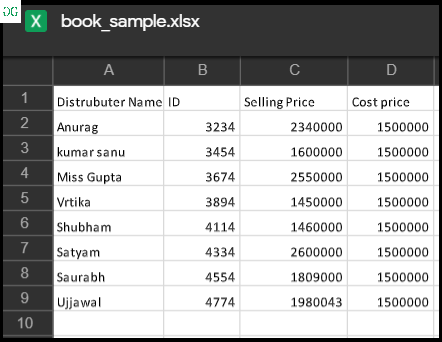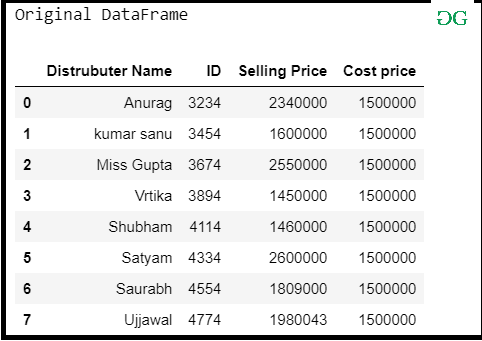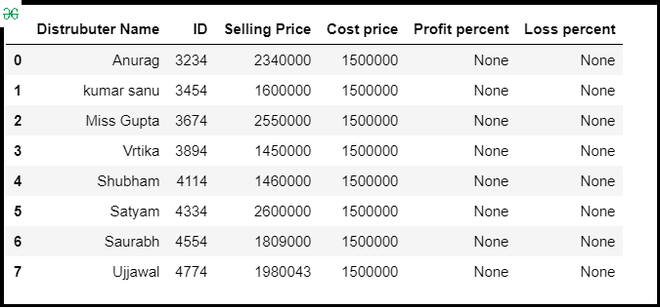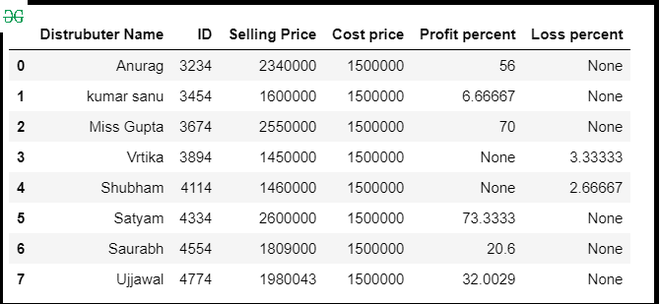# Find the profit and loss percent in the given Excel sheet using Pandas

• Last Updated : 05 Sep, 2020

In these articles, let’s discuss how to extract data from the Excel file and find the profit percent and loss percent at the given data. Suppose our Excel file looks like then we have to extract the Selling Price and Cost Price from the column and find the profit percent and loss percent and store it into a new DataFrame column.So, Let’s discuss the approach:

Step 1: Import the required module and read data from excel.

## Python3

 `# importing module`` ` `import` `pandas as pd`` ` `# Creating df``# Reading data from Excel``data ``=` `pd.read_excel(``"excel_work/book_sample.xlsx"``)``print``(``"Original DataFrame"``)``data`

Output :Step 2: Create a new column in DataFrame for store Profit percent and Loss percent.

## Python3

 `# Create column for profit and loss``data[``'Profit percent'``]``=` `None``data[``'Loss percent'``] ``=` `None``data`

Output :Step 3: Set index for selling price, Cost price, Profit percent, and Loss percent.

## Python3

 `# set index``index_selling ``=` `data.columns.get_loc(``'Selling Price'``)``index_cost ``=` `data.columns.get_loc(``'Cost price'``)``index_profit ``=` `data.columns.get_loc(``'Profit percent'``)``index_loss ``=` `data.columns.get_loc(``'Loss percent'``)`` ` `print``(index_selling, index_cost, index_profit, index_loss)`

Output :

```2 3 4 5
```

Step 4: Compute profit and loss percent according to there each column index.

```profit = (SP) - (CP)
profit % = (profit/ CP × 100)%
Loss = (CP) - (SP)
Loss % = (loss/ CP × 100)%```

## Python3

 `# Loop for accessing every index in DataFrame``# and compute Profit % and loss %``# and store into new column in DataFrame``for` `row ``in` `range``(``0``, ``len``(data)):``    ``if` `data.iat[row, index_selling] > data.iat[row, index_cost]:``        ``profit ``=` `data.iat[row, index_selling] ``-` `data.iat[row, index_cost]``        ``data.iat[row, index_profit] ``=` `(profit``/``data.iat[row, index_cost]``*``100``)`` ` `    ``else``:``        ``loss ``=` `abs``(data.iat[row, index_cost]``-``data.iat[row, index_selling])``        ``data.iat[row, index_loss] ``=` `(loss``/``data.iat[row, index_cost]``*``100``)`` ` `data`

Output :My Personal Notes arrow_drop_up